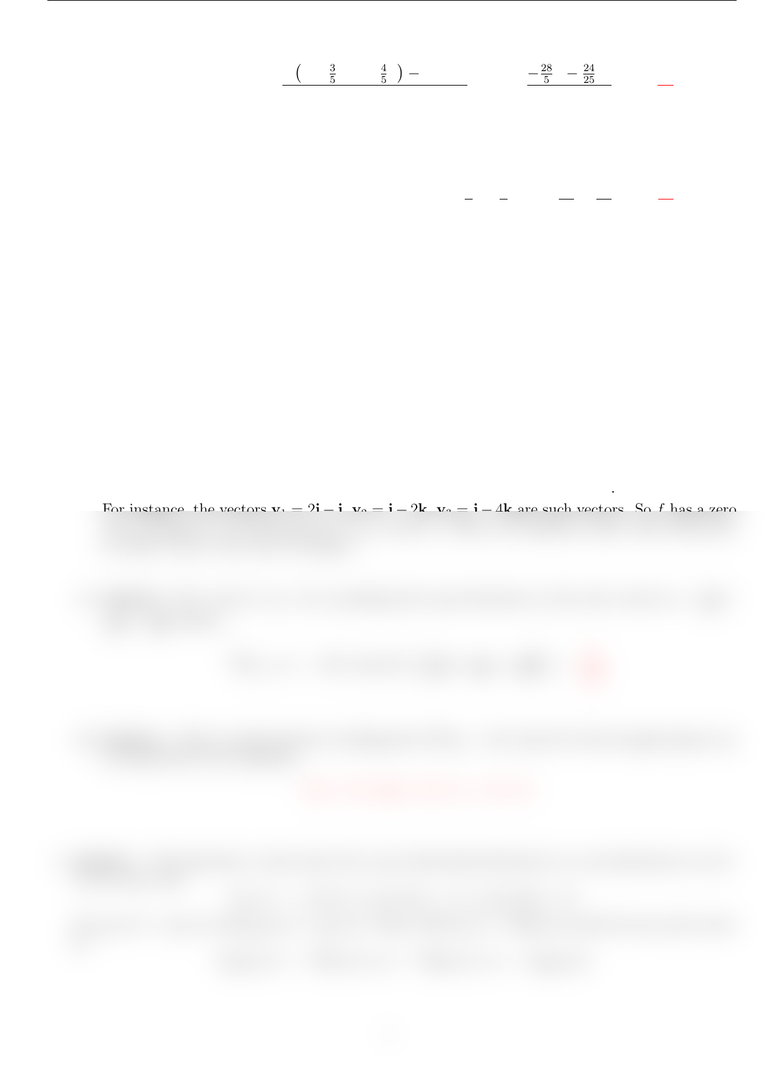# MTH 101 Chapter 7: dis 7 sol

16 views2 pages
School
Department
Course
ProfessorCalculus II Discussion Problems (Week 7) Solutions Spring 2021
Duf(1,2) = lim
h0
f1 + 3
5h, 2 + 4
5hf(1,2)
h= lim
h028
5h24
25 h2
h=28
5.
(b) Answer: Since f= (2y)i+ (2x6y)jand (f)(1,2) = 4i10j, we have
Duf(1,2) = (f)(1,2) ·u= (4i10j)·3
5i+4
5j=12
540
5=28
5.
2. Answer: It can be seen from the graph that fx(0,0) = fy(0,0) = 0. So (f)(0,0) = 0. Hence,
for any unit vector u,
(f)(0,0) ·u= 0.
i.e., the directional derivative of fat (0,0) is zero in every direction. In particular, it is zero in the
direction of 2i+jas well.
3. (a) Answer: At X0,fincreases most rapidly in the direction of f(X0) = 2i+ 4j+k.
(b) Answer: Since f(X0) = 2i+ 4j+k,fhas a zero rate of change in any direction orthogonal
to 2i+ 4j+k. Using dot product, we can easily ﬁnd vectors vsuch that v·(2i+ 4j+k) = 0.
For instance, the vectors v1= 2ij,v2=i2k,v3=j4kare such vectors. So fhas a zero
rate of change in the directions of v1,v2, and v3. (There are innitely many other directions
in which fhas a zero rate of change.)
(c) Answer: The vector i+jkis pointing the same direction as the unit vector u=1
3i+
1
3j1
3k. Hence
fX0·u= (2i+ 4j+k)·1
3i+1
3j1
3k=5
3.
(d) Answer: Such a tangent plane is orthogonal to fX0= 2i+ 4j+k. So the tangent plane can
be described by the equation
2(x1) + 4(y2) + (z4) = 0.
4. Answer: The functions fand ghave the same directional derivative in every direction at (a, b).
To see why, note
g(x, y) = f(a, b) + fx(a, b)(xa) + fy(a, b)(yb).
So gx(a, b) = fx(a, b) and gy(a, b) = fy(a, b). Thus, (f)(a, b) = (g)(a, b) and, for any unit vector
u,
Duf(a, b) = f(a, b)·u=g(a, b)·u=Dug(a, b).
1
Unlock document

This preview shows half of the first page of the document.
Unlock all 2 pages and 3 million more documents.